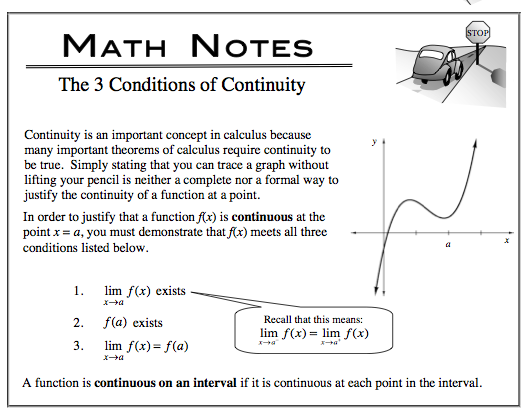### Home > CALC > Chapter 2 > Lesson 2.2.3 > Problem2-88

2-88.

Given $f ( x ) = \left\{ \begin{array} { c l } { x ^ { 2 } } & { \text { for } x < 1 , \text { but } x \neq - 1 } \\ { 3 } & { \text { for } x = 1 } \\ { 2 x - 1 } & { \text { for } 1 < x < 3 } \\ { 4 } & { \text { for } x \geq 3 } \end{array} \right.$

1. Sketch $f(x)$.

2. For what values of $x$ is $f(x)$ NOT continuous?Use the eTool below to view the parts of the graph.
Click the link to the right to view the full version of the eTool: Calc 2-88 HW eTool (Desmos)